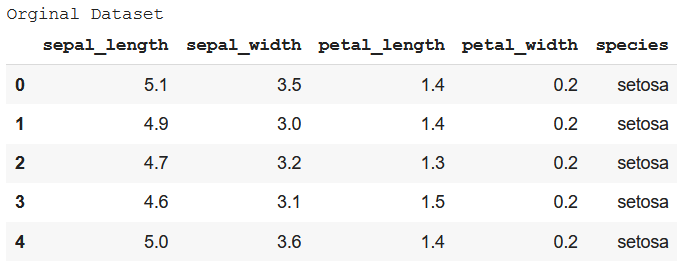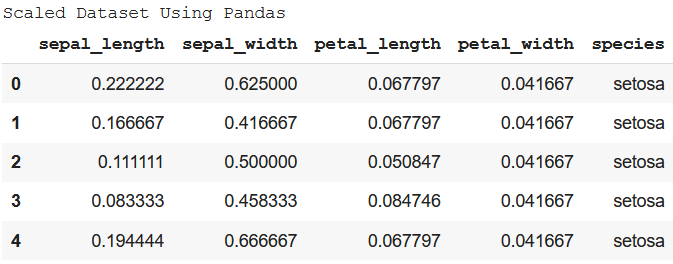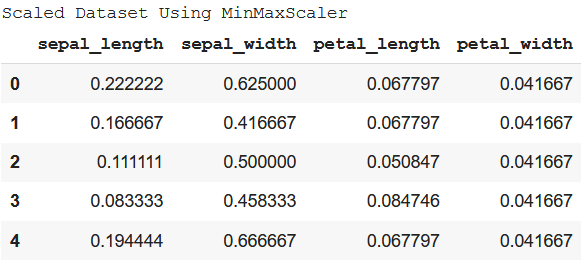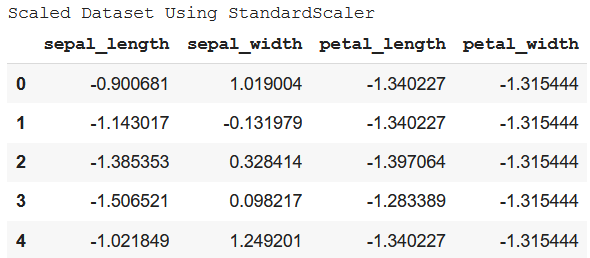Related Articles

# How to scale Pandas DataFrame columns ?

• Last Updated : 02 Jul, 2021

When a dataset has values of different columns at drastically different scales, it gets tough to analyze the trends and patterns and comparison of the features or columns. So, in cases where all the columns have a significant difference in their scales, are needed to be modified in such a way that all those values fall into the same scale. This process is called Scaling.

There are two most common techniques of how to scale columns of Pandas dataframe – Min-Max Normalization and Standardization. Both of them have been discussed in the content below.

Dataset in Use: Iris### Min-Max Normalization

Here, all the values are scaled in between the range of [0,1] where 0 is the minimum value and 1 is the maximum value. The formula for Min-Max Normalization is –Method 1: Using Pandas and Numpy

The first way of doing this is by separately calculate the values required as given in the formula and then apply it to the dataset.

Example:

## Python3

 import seaborn as snsimport pandas as pdimport numpy as np data = sns.load_dataset('iris')print('Original Dataset')data.head() # Min-Max Normalizationdf = data.drop('species', axis=1)df_norm = (df-df.min())/(df.max()-df.min())df_norm = pd.concat((df_norm, data.species), 1) print("Scaled Dataset Using Pandas")df_norm.head()

Output:Method 2: Using MinMaxScaler from sklearn

This is a straightforward method of doing the same. It just requires sklearn module to be imported.

Example:

## Python3

 import seaborn as snsfrom sklearn.preprocessing import MinMaxScalerimport pandas as pd data = sns.load_dataset('iris')print('Original Dataset')data.head() scaler = MinMaxScaler() df_scaled = scaler.fit_transform(df.to_numpy())df_scaled = pd.DataFrame(df_scaled, columns=[  'sepal_length', 'sepal_width', 'petal_length', 'petal_width']) print("Scaled Dataset Using MinMaxScaler")df_scaled.head()

Output:### Standardization

Standardization doesn’t have any fixed minimum or maximum value. Here, the values of all the columns are scaled in such a way that they all have a mean equal to 0 and standard deviation equal to 1. This scaling technique works well with outliers. Thus, this technique is preferred if outliers are present in the dataset.

Example:

## Python3

 import pandas as pdfrom sklearn.preprocessing import StandardScalerimport seaborn as sns data = sns.load_dataset('iris')print('Original Dataset')data.head() std_scaler = StandardScaler() df_scaled = std_scaler.fit_transform(df.to_numpy())df_scaled = pd.DataFrame(df_scaled, columns=[  'sepal_length','sepal_width','petal_length','petal_width']) print("Scaled Dataset Using StandardScaler")df_scaled.head()

Output :Attention geek! Strengthen your foundations with the Python Programming Foundation Course and learn the basics.

To begin with, your interview preparations Enhance your Data Structures concepts with the Python DS Course. And to begin with your Machine Learning Journey, join the Machine Learning – Basic Level Course

My Personal Notes arrow_drop_up